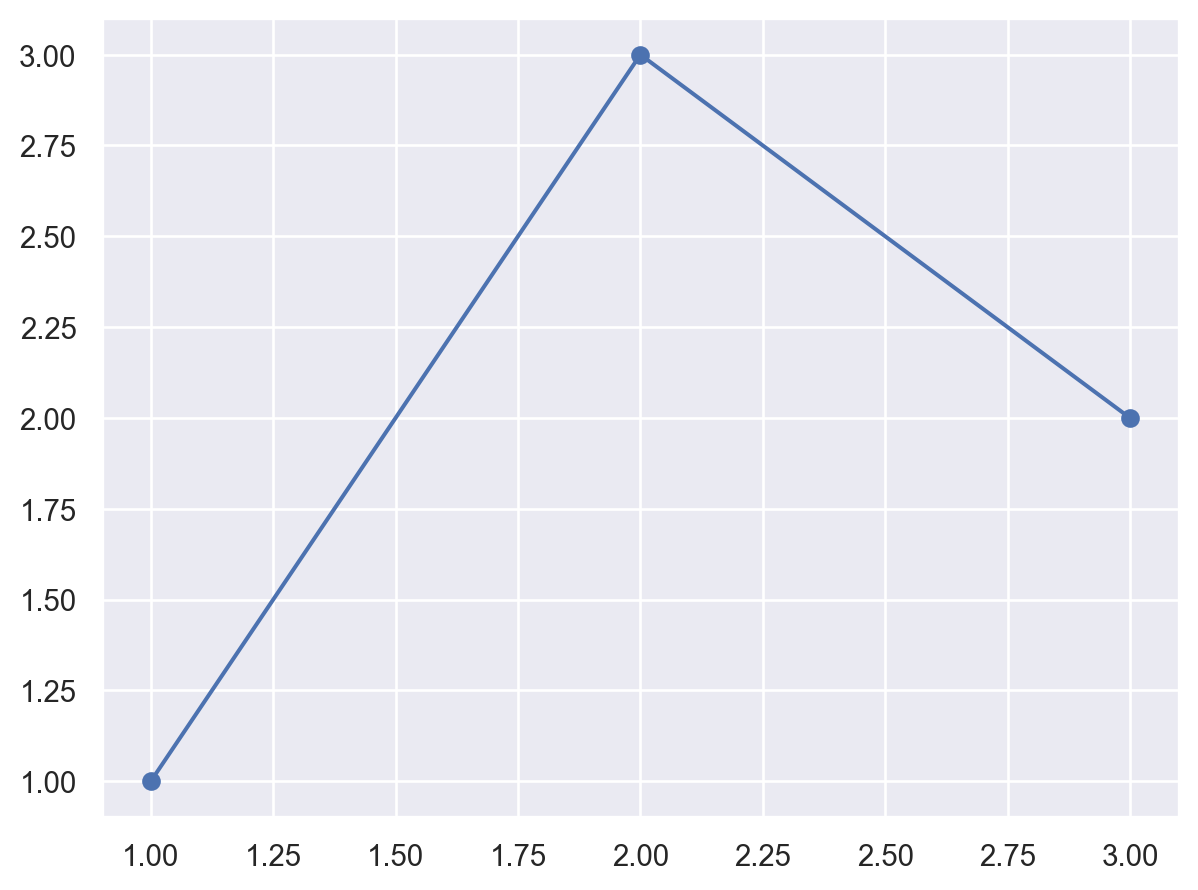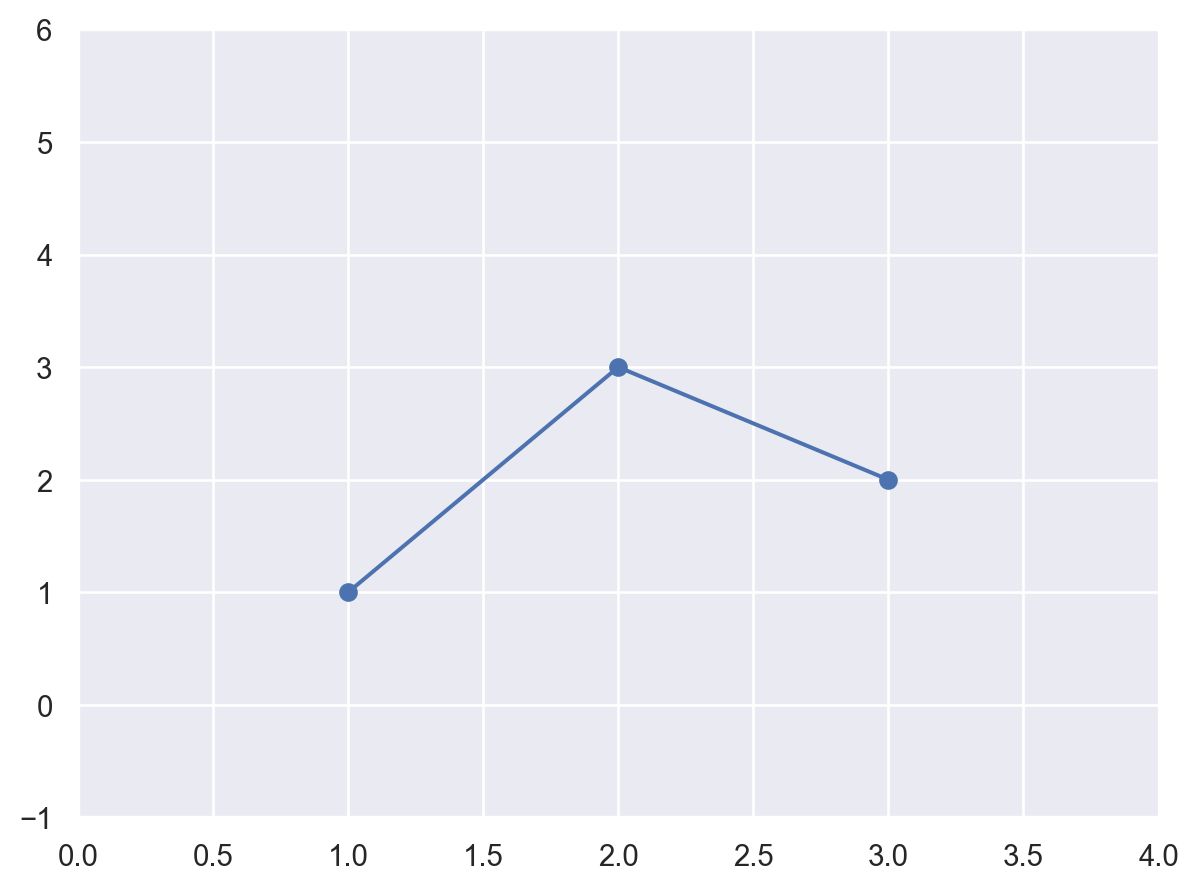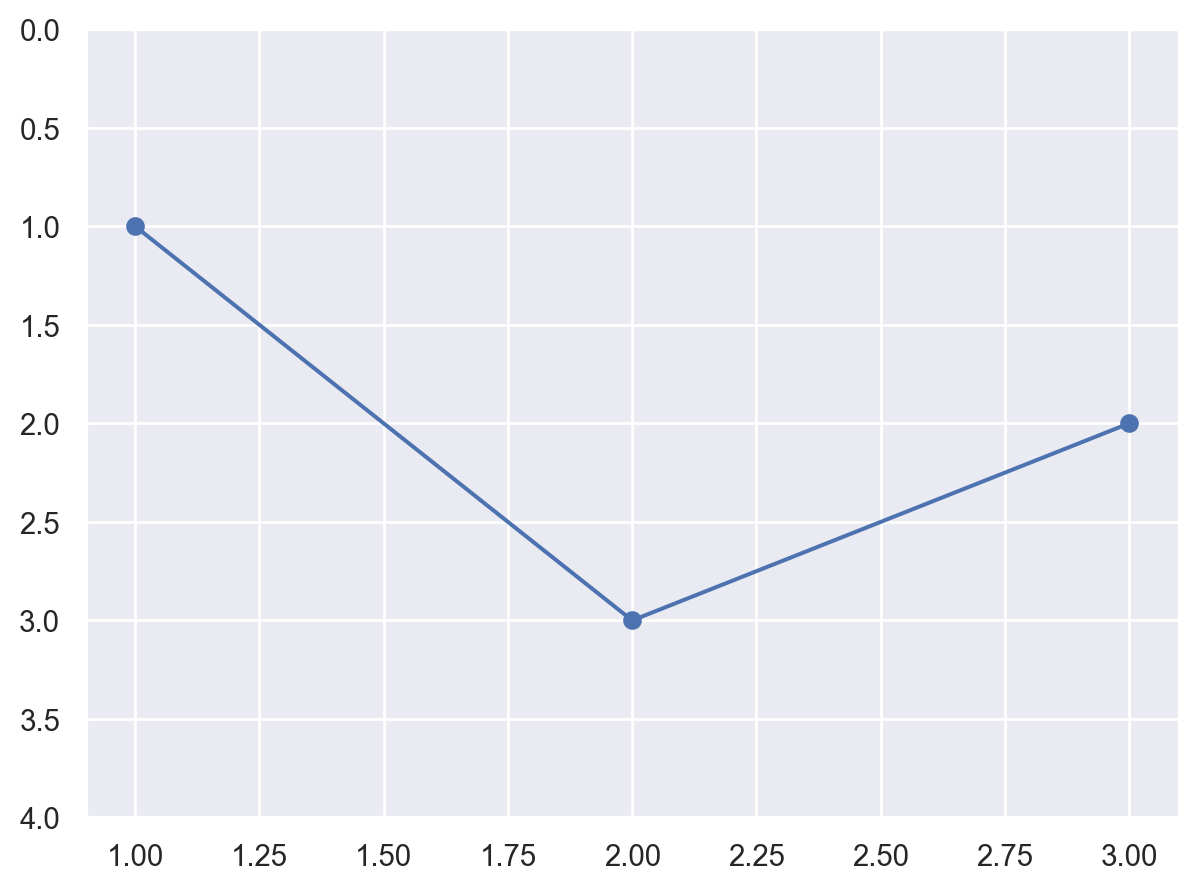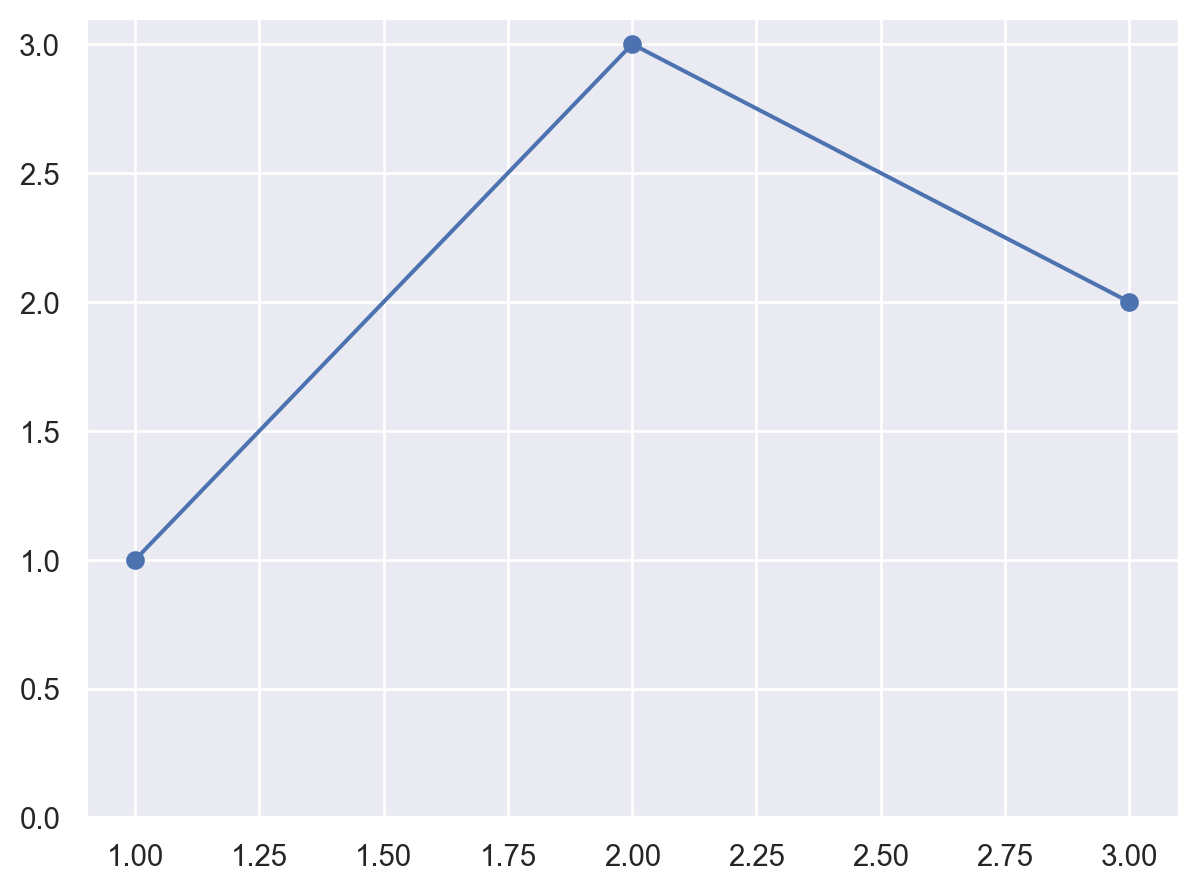# seaborn.objects.Plot.limit#

Plot.limit(**limits)#

Control the range of visible data.

Keywords correspond to variables defined in the plot, and values are a `(min, max)` tuple (where either can be `None` to leave unset).

Limits apply only to the axis; data outside the visible range are still used for any stat transforms and added to the plot.

Behavior for non-coordinate variables is currently undefined.

Examples

By default, plot limits are automatically set to provide a small margin around the data (controlled by `Plot.theme()` parameters `axes.xmargin` and `axes.ymargin`):

```p = so.Plot(x=[1, 2, 3], y=[1, 3, 2]).add(so.Line(marker="o"))
p
```Pass a `min`/`max` tuple to pin the limits at specific values:

```p.limit(x=(0, 4), y=(-1, 6))
```Reversing the `min`/`max` values will invert the axis:

```p.limit(y=(4, 0))
```Use `None` for either side to maintain the default value:

```p.limit(y=(0, None))
```